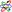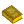## Symmetry

An intrinsic property of a mathematical object which causes it to remain invariant under certain classes of transformations (such as Rotation, Reflection, Inversion, or more abstract operations). The mathematical study of symmetry is systematized and formalized in the extremely powerful and beautiful area of mathematics called Group Theory.

Symmetry can be present in the form of coefficients of equations as well as in the physical arrangement of objects. By classifying the symmetry of polynomial equations using the machinery of Group Theory, for example, it is possible to prove the unsolvability of the general Quintic Equation.

In physics, an extremely powerful theorem of Noether states that each symmetry of a system leads to a physically conserved quantity. Symmetry under Translation corresponds to momentumconservation, symmetry under Rotation to angular momentumconservation, symmetry in time to energyconservation, etc.

ReferencesSymmetry

Eppstein, D. ``Symmetry and Group Theory.'' http://www.ics.uci.edu/~eppstein/junkyard/sym.html.

Farmer, D. Groups and Symmetry. Providence, RI: Amer. Math. Soc., 1995.

Pappas, T. ``Art & Dynamic Symmetry.'' The Joy of Mathematics. San Carlos, CA: Wide World Publ./Tetra, pp. 154-155, 1989.

Rosen, J. Symmetry in Science: An Introduction to the General Theory. New York: Springer-Verlag, 1995.

Schattschneider, D. Visions of Symmetry: Notebooks, Periodic Drawings, and Related Work of M. C. Escher. New York: W. H. Freeman, 1990.

Stewart, I. and Golubitsky, M. Fearful Symmetry. New York: Viking Penguin, 1993.